# Simultaneously Fitting Source and Background Spectra## Overview

#### Synopsis:

If you would like to fit a background-subtracted source spectrum using a common RMF and ARF for source and background, simply read the source spectrum fits file into Sherpa, subtract the background, and fit it. To fit source and background spectra simultaneously with proper and distinct RMFs and ARFs, load the source and background as different data sets. This thread illustrates both cases.

The sample data files used in this thread are available in sherpa.tar.gz; they can be generated by following the CIAO thread Extract ACIS Spectra and Response Files for Pointlike Sources.

Last Update: 24 Mar 2022 - revised for CIAO 4.14, no new content change.

## Statistical Issues: Background Subtraction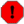A typical dataset may contain multiple spectra, one of which contains contributions from the source and the background, and one or more which contain background counts alone. (The background itself may contain contributions from the cosmic X-ray background, the particle background, and so on, but we ignore this complication.)

The proper way to treat background data is to model them. However, many X-ray astronomers subtract background data from the raw data.

Why should one not subtract background?

• It reduces the amount of statistical information in the analysis—the final fit parameter values will be a less accurate estimate of the true values.
• The background-subtracted data are not Poisson-distributed; one cannot fit them with the Poisson likelihood or the Cash statistic, even in the low-counts limit. For example, subtracting a background can give negative counts; this is definitely not Poissonian!
• Fluctuations, particularly in the vicinity of localized features, can adversely affect analysis.

## Fit a Background-Subtracted Source

### Reading FITS data for source and background

By default, specextract updates the header keywords BACKFILE, RESPFILE, and ANCRFILE in the source spectrum file:

unix% dmkeypar 3c273.pi BACKFILE echo+
3c273_bg.pi

unix% dmkeypar 3c273.pi RESPFILE echo+
3c273.rmf

unix% dmkeypar 3c273.pi ANCRFILE echo+
3c273.arf


On account of these keywords, Sherpa automatically reads in the (ungrouped) background spectrum "3c273_bg.pi" and the source response matrices "3c273.rmf" and "3c273.arf" when the source spectrum is read:

sherpa> load_pha("3c273.pi")
statistical errors were found in file '3c273.pi'
but not used; to use them, re-read with use_errors=True
statistical errors were found in file '3c273_bg.pi'
but not used; to use them, re-read with use_errors=TrueIf Sherpa does not automatically read in the background data, then it can be input as follows:

sherpa> load_bkg("3c273_bg.pi")
statistical errors were found in file '3c273_bg.pi'
but not used; to use them, re-read with use_errors=True


### Subtracting the background

The user can then subtract the background from the source spectrum and fit the background-subtracted source spectrum using response matrices for the source only. The following commands may be used as an example script for subtracting the background and then visualizing the 0.3-3 keV background-subtracted source data in Sherpa:

sherpa> subtract()
sherpa> set_xlog()
sherpa> set_ylog()
sherpa> plot_data()


### Figure 1: The background-subtracted source spectrum: all the data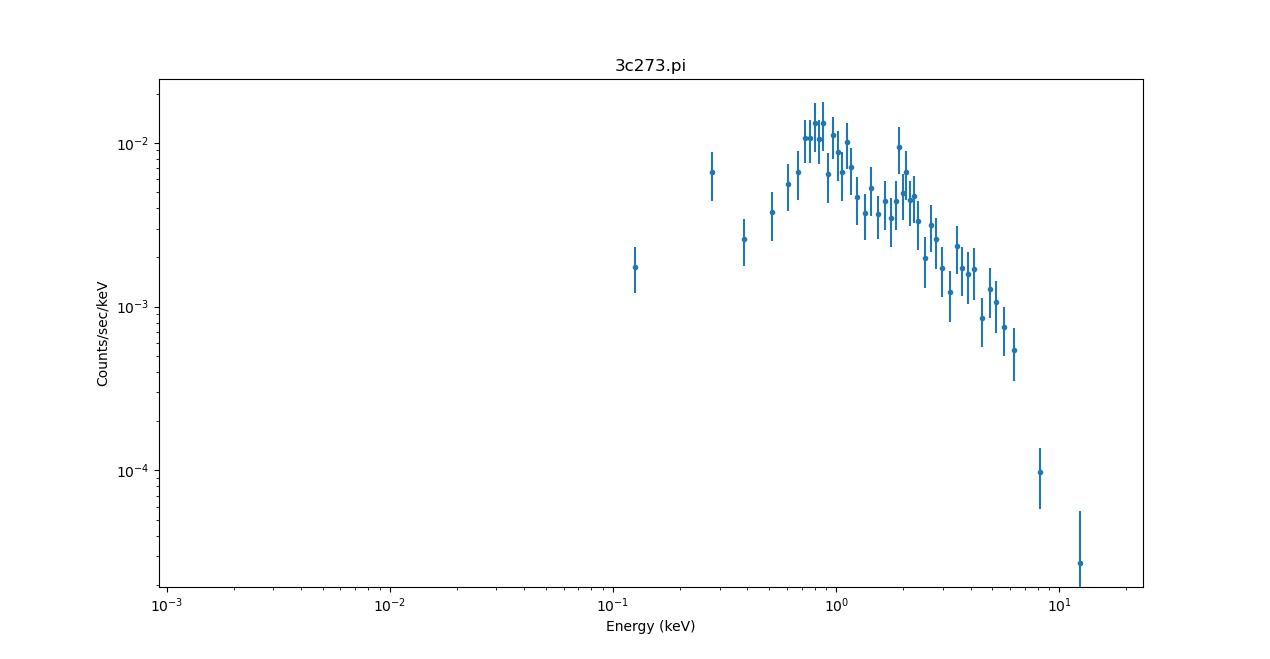### Figure 1: The background-subtracted source spectrum: all the data

Plot of background-subtracted spectrum of 3C 273 for the full energy range. The axes are both drawn with a log scale because of the calls to set_xlog and set_ylog.

The PHA data plots display the full data range of the noticed data, so in this case the x-axis shows data down to $$10^{-3}$$ keV, as the e_min values from the RMF are used for this plot:

sherpa> print(get_rmf().e_min)
0.0014600001741200686


Applying the 0.3-3 keV energy filter:

sherpa> notice(0.3, 3.)
sherpa> plot_data(xlog=False, ylog=False)


produces Figure 2.

### Figure 2: The background-subtracted source spectrum: 0.3 - 3 keV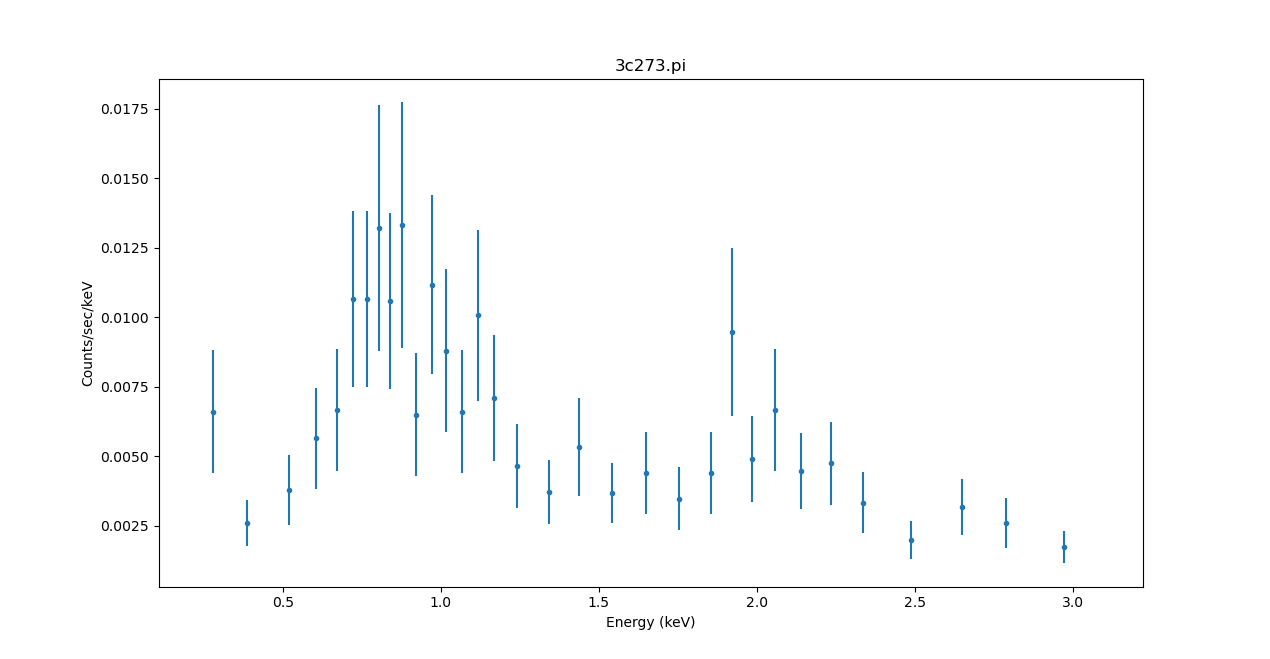### Figure 2: The background-subtracted source spectrum: 0.3 - 3 keV

Plot of background-subtracted spectrum of 3C 273, with linear axes for the energy range 0.3 to 3 keV.

The get_bkg_scale function—not to be confused with get_backscal—returns the value of the coefficient which is used to scale background counts during background subtraction of a source spectrum, or a simultaneous fit of source and background spectra. The complete scaling factor used to scale the background counts depends on how the background is handled. When subtracting the background, which corresponds to the default units='counts' option, the ratio is the product of the source-to-background exposure and backscale (extraction region area).

The background-subtracted spectrum is then:

$\mathrm{spectrum_{src-bkg}\ [cts/s]} = \frac{\mathrm{counts_{src}}}{\mathrm{exposure_{src}}} - \left( \frac{\mathrm{counts_{bkg}}}{\mathrm{exposure_{bkg}}} \right) \left( \frac{\mathrm{backscale_{src}}}{\mathrm{backscale_{bkg}}} \right)$

and the background scaling factor is:

$\mathrm{background\ scaling\ factor} = \left( \frac{\mathrm{exposure_{src}}}{\mathrm{exposure_{bkg}}} \right) \left( \frac{\mathrm{backscale_{src}}}{\mathrm{backscale_{bkg}}} \right).$

If the background is to be modelled, rather than subtracted, we only want the ratio of the BACKSCALE values, which is provided by the (new to CIAO 4.13) option: units='rate'. This is discussed in the Manually defining source and background models section below.Note

The get_backscal function returns the value associated with the OGIP PHA header keyword BACKSCAL, which is only one component of the complete scaling factor.

The background scaling factor is also returned by the show_data and show_all functions. The value returned by the get_bkg_scale function can be checked by doing the following in Sherpa:

sherpa> print(get_bkg_scale())
0.134920643888096


which can be compared to

sherpa> t1 = get_exposure() * get_backscal()
sherpa> t2 = get_exposure(bkg_id=1) / get_backscal(bkg_id=1)
sherpa> manual_bkg_scale_check = t1 / t2
sherpa> print(manual_bkg_scale_check)
0.134920643888096


### Defining the source instrument response

Since the header of the input source spectrum file referenced the instrument response files, an instrument response was automatically defined:

sherpa> print(get_arf())
name     = 3c273.arf
energ_lo = Float64
energ_hi = Float64
specresp = Float64
bin_lo   = None
bin_hi   = None
exposure = 38570.69248122
ethresh  = 1e-10

sherpa> print(get_rmf())
name     = 3c273.rmf
detchans = 1024
energ_lo = Float64
energ_hi = Float64
n_grp    = UInt64
f_chan   = UInt32
n_chan   = UInt32
matrix   = Float64
offset   = 1
e_min    = Float64
e_max    = Float64
ethresh  = 1e-10

sherpa> print(get_bkg_arf())
name     = 3c273.arf
energ_lo = Float64
energ_hi = Float64
specresp = Float64
bin_lo   = None
bin_hi   = None
exposure = 38570.69248122
ethresh  = 1e-10

sherpa> print(get_bkg_rmf())
name     = 3c273.rmf
detchans = 1024
energ_lo = Float64
energ_hi = Float64
n_grp    = UInt64
f_chan   = UInt32
n_chan   = UInt32
matrix   = Float64
offset   = 1
e_min    = Float64
e_max    = Float64
ethresh  = 1e-10


Note that the instrument response was automatically set up for both the source and background. However, unless a background model is defined for fitting using the set_bkg_model command, then the background data will be ignored.If you manually read in a background file after reading the source data, the background instrument response will be reset. To set it to be identical to the source instrument response, use load_bkg_arf and load_bkg_rmf with the source response files:

sherpa> load_bkg_rmf("3c273.rmf")

sherpa> print(get_bkg_arf())
name     = 3c273.arf
energ_lo = Float64
energ_hi = Float64
specresp = Float64
bin_lo   = None
bin_hi   = None
exposure = 38570.69248122
ethresh  = 1e-10

sherpa> print(get_bkg_rmf())
name     = 3c273.rmf
detchans = 1024
energ_lo = Float64
energ_hi = Float64
n_grp    = UInt64
f_chan   = UInt32
n_chan   = UInt32
matrix   = Float64
offset   = 1
e_min    = Float64
e_max    = Float64
ethresh  = 1e-10


### Defining and fitting a source model

The following commands may be used as an example script for fitting the background-subtracted data with a source model consisting of an absorbed power-law:

sherpa> set_source(xsphabs.abs1 * powlaw1d.p1)
sherpa> show_source()
Model: 1
(xsphabs.abs1 * powlaw1d.p1)
Param        Type          Value          Min          Max      Units
-----        ----          -----          ---          ---      -----
abs1.nH      thawed            1            0       100000 10^22 atoms / cm^2
p1.gamma     thawed            1          -10           10
p1.ref       frozen            1 -3.40282e+38  3.40282e+38
p1.ampl      thawed            1            0  3.40282e+38

sherpa> abs1.nh = 0.1
sherpa> guess(p1)
sherpa> print(p1)
powlaw1d.p1
Param        Type          Value          Min          Max      Units
-----        ----          -----          ---          ---      -----
p1.gamma     thawed            1          -10           10
p1.ref       frozen            1 -3.40282e+38  3.40282e+38
p1.ampl      thawed  0.000143003  1.43003e-07     0.143003

sherpa> fit()
Dataset               = 1
Method                = levmar
Statistic             = chi2gehrels
Initial fit statistic = 89.6271
Final fit statistic   = 15.474 at function evaluation 184
Data points           = 33
Degrees of freedom    = 30
Probability [Q-value] = 0.986773
Reduced statistic     = 0.515801
Change in statistic   = 74.1531
abs1.nH        0.000216916  +/- 0.0160164
p1.gamma       1.70304      +/- 0.155556
p1.ampl        0.000160838  +/- 1.56648e-05

sherpa> plot_fit_resid()


The results are shown in Figure 3.

### Figure 3: Fit of the background-subtracted data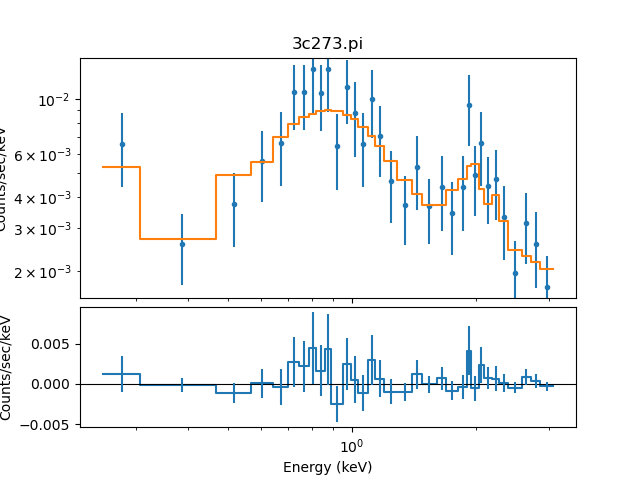### Figure 3: Fit of the background-subtracted data

Plot of the source model fit to the background-subtracted spectrum of 3C 273 and residuals.

The confidence function may be used to estimate the errors on the individual parameters of the fit; in this example, we choose to explicitly calculate the 1σ errors (although it is also the default setting):

sherpa> get_conf_opt()
{'sigma': 1,
'eps': 0.01,
'maxiters': 200,
'soft_limits': False,
'remin': 0.01,
'fast': False,
'parallel': True,
'numcores': 4,
'maxfits': 5,
'max_rstat': 3,
'tol': 0.2,
'verbose': False,
'openinterval': False}

sherpa> set_conf_opt("sigma", 1)
sherpa> conf()
abs1.nH lower bound:    -----
abs1.nH upper bound:    0.0117216
p1.gamma lower bound:   -0.105005
p1.gamma upper bound:   0.125461
p1.ampl lower bound:    -8.25981e-06
p1.ampl upper bound:    1.46135e-05
Dataset               = 1
Confidence Method     = confidence
Iterative Fit Method  = None
Fitting Method        = levmar
Statistic             = chi2gehrels
confidence 1-sigma (68.2689%) bounds:
Param            Best-Fit  Lower Bound  Upper Bound
-----            --------  -----------  -----------
abs1.nH       0.000216916        -----    0.0117216
p1.gamma          1.70304    -0.105005     0.125461
p1.ampl       0.000160838 -8.25981e-06  1.46135e-05


## Simultaneously Fit Source and Background with the Same Responses

Instead of subtracting the background, the user may choose to simultaneously fit the source and background spectra, using the same (source) RMF and ARF.

### Reading FITS data for source and background

Again, Sherpa automatically reads in the (ungrouped) background spectrum and the source response matrices when the source spectrum is read:

sherpa> clean()
statistical errors were found in file '3c273.pi'
but not used; to use them, re-read with use_errors=True
statistical errors were found in file '3c273_bg.pi'
but not used; to use them, re-read with use_errors=True


### Defining the source and background instrument response

Since the header of the input source spectrum file referenced the instrument response files, an instrument response was automatically defined:

sherpa> print(get_arf())
name     = 3c273.arf
energ_lo = Float64
energ_hi = Float64
specresp = Float64
bin_lo   = None
bin_hi   = None
exposure = 38570.69248122
ethresh  = 1e-10

sherpa> print(get_rmf())
name     = 3c273.rmf
detchans = 1024
energ_lo = Float64
energ_hi = Float64
n_grp    = UInt64
f_chan   = UInt32
n_chan   = UInt32
matrix   = Float64
offset   = 1
e_min    = Float64
e_max    = Float64
ethresh  = 1e-10

sherpa> print(get_bkg_arf())
name     = 3c273.arf
energ_lo = Float64
energ_hi = Float64
specresp = Float64
bin_lo   = None
bin_hi   = None
exposure = 38570.69248122
ethresh  = 1e-10

sherpa> print(get_bkg_rmf())
name     = 3c273.rmf
detchans = 1024
energ_lo = Float64
energ_hi = Float64
n_grp    = UInt64
f_chan   = UInt32
n_chan   = UInt32
matrix   = Float64
offset   = 1
e_min    = Float64
e_max    = Float64
ethresh  = 1e-10


The instrument response was automatically set up for both the source and background. However, unless a background model is defined for fitting using the set_bkg_model command, then the background data will be ignored. In order to fit the background simultaneously with the source, the set_bkg_model() command will be issued in the next step.

As with the Defining the source instrument response section, the load_bkg_arf and load_bkg_rmf routines can be used to set the responses.

The same data range is used as previously:

sherpa> notice(0.3, 3)
sherpa> plot("data", "bkg")


### Figure 4: Comparing the source and background datasets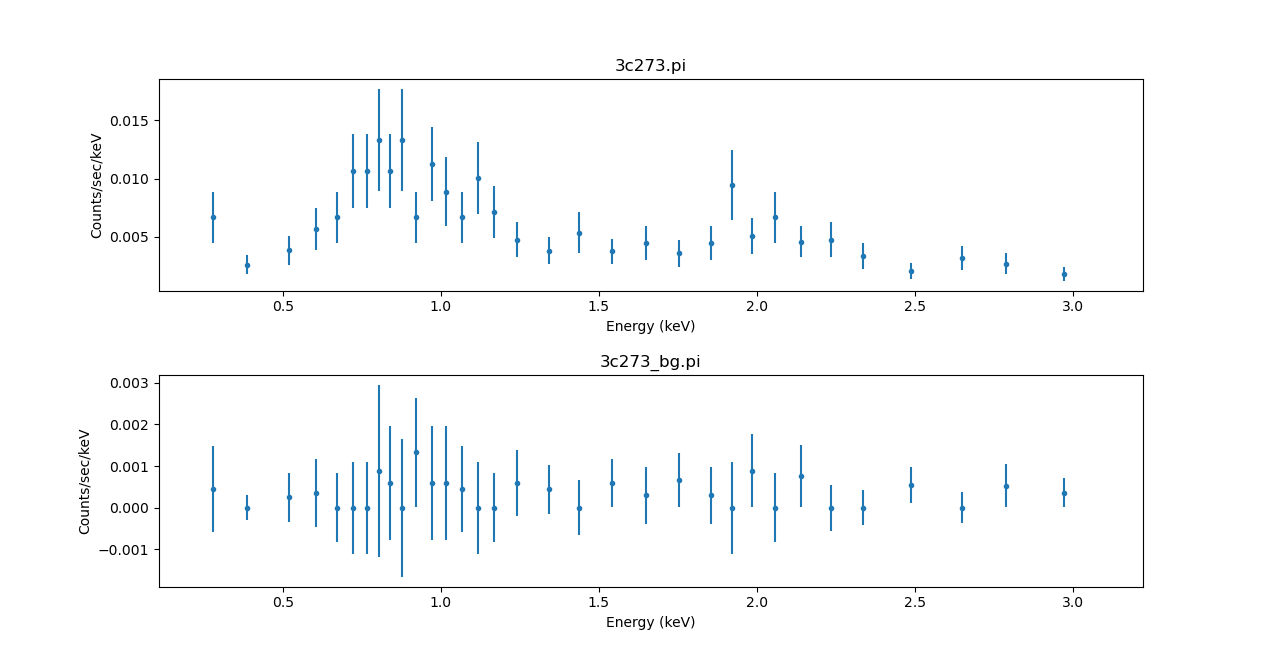### Figure 4: Comparing the source and background datasets

The plot function is used to display both the data (plot_data) and background (plot_bkg). Note that the default is for the background to use the same grouping as the source dataset: as normally the background has less counts than the source this may lead to low-significance points.

### Re-grouping the data and background

In this section we are going to re-group the data so that:

The first step is to remove both the grouping and any data filters:

sherpa> ungroup()
sherpa> notice()


We can now apply the filter to the ungrouped data and store the mask arrays for both source and background datasets (this isn't needed in this example as they are the same, but if we had used a different energy range they would differ):

sherpa> notice(0.3, 3)


The mask arrays can then be used in a call to group_counts, noting that we need to invert them with the ~ operator for the tabStops argument:

sherpa> group_counts(20, tabStops=~smask)
sherpa> plot_data(ylog=True)
sherpa> plot_bkg(overplot=True)


### Figure 5: Changing the grpuping for the background dataset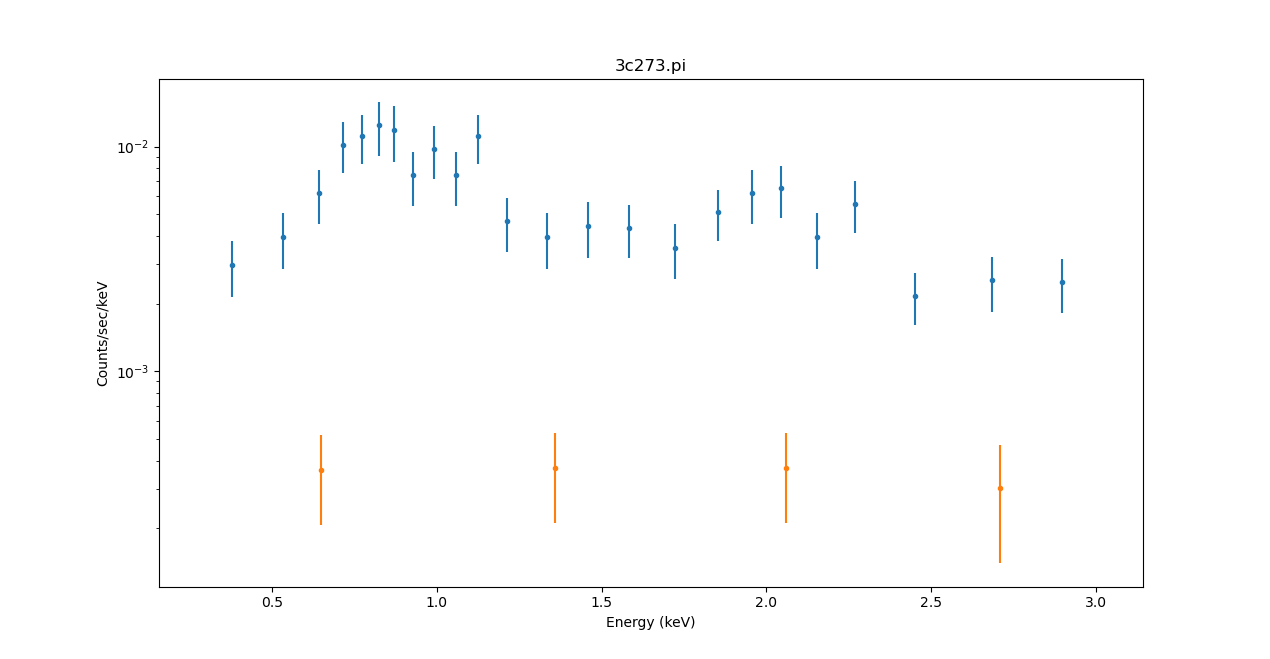### Figure 5: Changing the grpuping for the background dataset

The orange points mark the background values. The reason for using such a low value of 10 counts per bin for the background is because there are not many counts in the background!

Although the thread started with calls to set_xlog and set_ylog, the call to clean earlier has meant that these settings have been cleared, hence the use of ylog in the plot_data call.Warning

The data as plotted does not account for any scaling factor between the source and background regions (that is, the relative BACKSCAL values of the two).

### Defining and fitting source and background models

The fit_bkg function is available (and the equivalent 'bkg_only' option of the fit command) allows you to fit models to just the background components. It fits the defined background model(s) to the background data set(s) by ID. It may be called with no arguments, in which case a fit is done simultaneously on all background data sets for which the user has defined a model to be fit; source data sets ready for fitting will be ignored in this case.

Here, we choose to simultaneously fit the source and background with the fit function, using an absorbed power-law model for the source and an unabsorbed power-law model for the background. The source and background models are defined using the set_source and set_bkg_model commands, respectively.

sherpa> set_source(xsphabs.abs1 * powlaw1d.srcp1)
sherpa> set_bkg_model(abs1 * powlaw1d.bkgp1)
sherpa> abs1.nh = 0.1
sherpa> guess(srcp1)
sherpa> guess(bkgp1)


We can view the model expressions with show_bkg_model and show_model. The latter now includes the background model and the scaling factor for the source and background apertures:

sherpa> show_bkg_model()
Background Model: 1:1
apply_rmf(apply_arf((38564.608926889 * (xsphabs.abs1 * powlaw1d.bkgp1))))
Param        Type          Value          Min          Max      Units
-----        ----          -----          ---          ---      -----
abs1.nH      thawed          0.1            0       100000 10^22 atoms / cm^2
bkgp1.gamma  thawed            1          -10           10
bkgp1.ref    frozen            1 -3.40282e+38  3.40282e+38
bkgp1.ampl   thawed  0.000161569  1.61569e-07     0.161569

sherpa> show_model()
Model: 1
apply_rmf(apply_arf((38564.608926889 * ((xsphabs.abs1 * powlaw1d.srcp1) + (0.13492064388809602 * (xsphabs.abs1 * powlaw1d.bkgp1))))))
Param        Type          Value          Min          Max      Units
-----        ----          -----          ---          ---      -----
abs1.nH      thawed          0.1            0       100000 10^22 atoms / cm^2
srcp1.gamma  thawed            1          -10           10
srcp1.ref    frozen            1 -3.40282e+38  3.40282e+38
srcp1.ampl   thawed  0.000161569  1.61569e-07     0.161569
bkgp1.gamma  thawed            1          -10           10
bkgp1.ref    frozen            1 -3.40282e+38  3.40282e+38
bkgp1.ampl   thawed  0.000161569  1.61569e-07     0.161569


We can start by fitting just the background components:

sherpa> fit_bkg()
Dataset               = 1
Method                = levmar
Statistic             = chi2gehrels
Initial fit statistic = 3707.75
Final fit statistic   = 0.180364 at function evaluation 242
Data points           = 4
Degrees of freedom    = 1
Probability [Q-value] = 0.671061
Reduced statistic     = 0.180364
Change in statistic   = 3707.57
abs1.nH        7.71392e-05  +/- 0.392598
bkgp1.gamma    1.1984       +/- 1.54133
bkgp1.ampl     1.05581e-05  +/- 1.23901e-05


The results could be inspected with plot_bkg_fit but let's go straight to fitting both the source and background datasets:

sherpa> fit()
Dataset               = 1
Method                = levmar
Statistic             = chi2gehrels
Initial fit statistic = 84.8399
Final fit statistic   = 13.941 at function evaluation 353
Data points           = 28
Degrees of freedom    = 23
Probability [Q-value] = 0.928549
Reduced statistic     = 0.60613
Change in statistic   = 70.8989
abs1.nH        0.000334765  +/- 0.04727
srcp1.gamma    1.67987      +/- 0.250904
srcp1.ampl     0.000163328  +/- 2.88748e-05
bkgp1.gamma    1.19428      +/- 0.548969
bkgp1.ampl     1.07525e-05  +/- 3.46457e-06

sherpa> plot_fit_delchi(ylog=True)
sherpa> plot_bkg_fit_delchi(overplot=True)


The results are shown Figure 6.

### Figure 6: Simultaneous fit of the source and background data (same responses)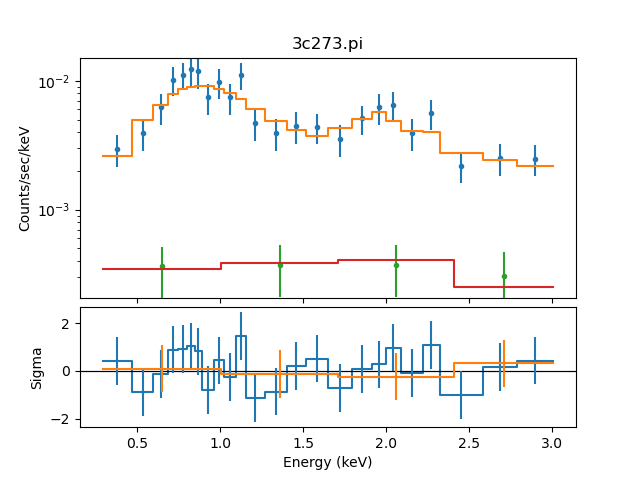### Figure 6: Simultaneous fit of the source and background data (same responses)

Plot of the simultaneous fit of the 3C 273 source and background spectra.

The calc_stat_info command (see also get_stat_info) may be used to access the goodness-of-fit statistics for the individual source and background data set included in the simultaneous fit, plus the simultaneous fit, without having to re-run the fit.

sherpa> calc_stat_info()
Dataset               = 1
Statistic             = chi2gehrels
Fit statistic value   = 13.7514
Data points           = 24
Degrees of freedom    = 19
Probability [Q-value] = 0.797984
Reduced statistic     = 0.723759

Background 1 in Dataset = 1
Statistic             = chi2gehrels
Fit statistic value   = 0.189552
Data points           = 4
Degrees of freedom    = 1
Probability [Q-value] = 0.66329
Reduced statistic     = 0.189552

Dataset               = 1
Statistic             = chi2gehrels
Fit statistic value   = 13.941
Data points           = 28
Degrees of freedom    = 23
Probability [Q-value] = 0.928549
Reduced statistic     = 0.60613


The confidence 1σ error estimates on the individual parameters of the fit are:

sherpa> conf()
abs1.nH lower bound:    -----
abs1.nH upper bound:    0.0413698
bkgp1.gamma lower bound:        -0.59936
bkgp1.gamma upper bound:        0.515119
bkgp1.ampl lower bound: -3.5308e-06
bkgp1.ampl upper bound: 3.07927e-06
srcp1.gamma lower bound:        -0.111196
srcp1.gamma upper bound:        0.222207
srcp1.ampl lower bound: -9.77831e-06
srcp1.ampl upper bound: 2.78773e-05
Dataset               = 1
Confidence Method     = confidence
Iterative Fit Method  = None
Fitting Method        = levmar
Statistic             = chi2gehrels
confidence 1-sigma (68.2689%) bounds:
Param            Best-Fit  Lower Bound  Upper Bound
-----            --------  -----------  -----------
abs1.nH       0.000334765        -----    0.0413698
srcp1.gamma       1.67987    -0.111196     0.222207
srcp1.ampl    0.000163328 -9.77831e-06  2.78773e-05
bkgp1.gamma       1.19428     -0.59936     0.515119
bkgp1.ampl    1.07525e-05  -3.5308e-06  3.07927e-06


## Simultaneously Fit Source and Background with Independent Responses

Instead of subtracting the background, the user may choose to simultaneously fit the source and background spectra, each with its own RMF and ARF.

### Reading FITS data for source and background

In this thread, we wish to fit spectral data from the FITS (ungrouped) data files source.pi and back.pi. These data sets are input into Sherpa with load_* commands:

sherpa> clean()

statistical errors were found in file 'source.pi'
but not used; to use them, re-read with use_errors=True

statistical errors were found in file 'source.pi'
but not used; to use them, re-read with use_errors=True


The source and background data sets can be plotted in separate windows with plot_data and plot_bkg, or together in one window with the following syntax:

sherpa> plot("data", "bkg")


The plots are displayed in Figure 7. The data are plotted in bin (i.e. channel) space, since we have not yet defined the instrument response (RMFs and ARFs).

### Figure 7: Plot of the ungrouped source and background data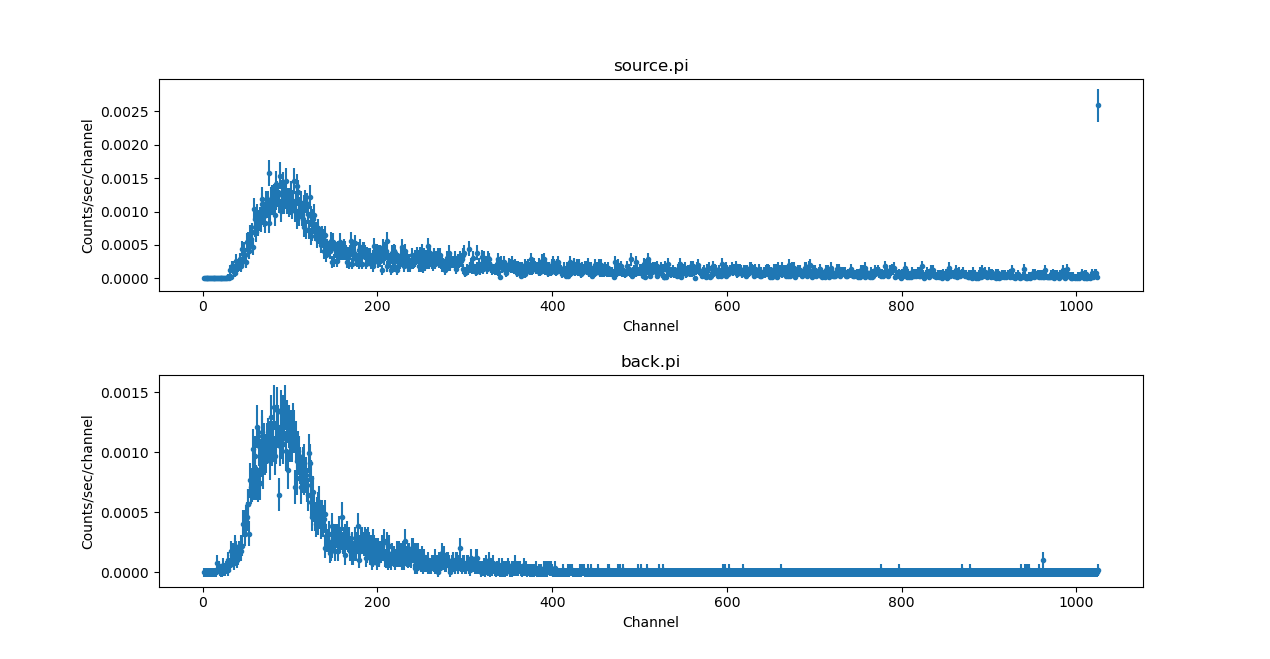### Figure 7: Plot of the ungrouped source and background data

Since no instrument responses have been loaded, the x-axis units are in channels rather then energy.

### Defining source and background instrument responses

Here we establish 1-D instrument responses by loading the source and background response files with load_* commands:

sherpa> load_arf("source.arf")

sherpa> load_bkg_arf("back.arf")


Now when we plot the data the x-axis is in energy, rather than channel (Figure 8):

sherpa> plot('data', 'bkg', xlog=True)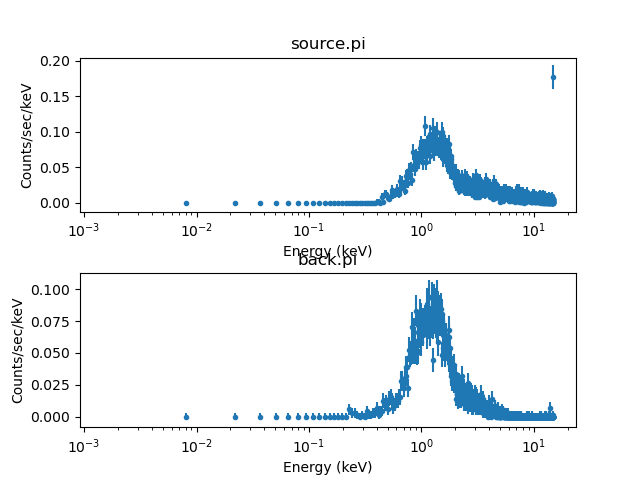Starting with CIAO 4.13, the ability to apply options to all the plots in a call to plot.

The current definition of the instrument response may be examined using show_all or get commands:

sherpa> print(get_arf())
name     = source.arf
energ_lo = Float64
energ_hi = Float64
specresp = Float64
bin_lo   = None
bin_hi   = None
exposure = 49470.94775939
ethresh  = 1e-10

sherpa> print(get_rmf())
name     = source.rmf
detchans = 1024
energ_lo = Float64
energ_hi = Float64
n_grp    = UInt64
f_chan   = UInt32
n_chan   = UInt32
matrix   = Float64
offset   = 1
e_min    = Float64
e_max    = Float64
ethresh  = 1e-10

sherpa> print(get_bkg_arf())
name     = back.arf
energ_lo = Float64
energ_hi = Float64
specresp = Float64
bin_lo   = None
bin_hi   = None
exposure = 49470.94775939
ethresh  = 1e-10

sherpa> print(get_bkg_rmf())
name     = back.rmf
detchans = 1024
energ_lo = Float64
energ_hi = Float64
n_grp    = UInt64
f_chan   = UInt32
n_chan   = UInt32
matrix   = Float64
offset   = 1
e_min    = Float64
e_max    = Float64
ethresh  = 1e-10


The output shows that source.arf and source.rmf currently define the source instrument response.

### Defining and fitting source and background models

We now need to define a source model for our source data set and a background model for our background data set. For these data, we find that absorbed blackbody spectra are appropriate. We define a model which includes an absorbing column and a blackbody for both the source and background:

sherpa> set_source(xsphabs.a1 * xsbbody.b1)
sherpa> set_bkg_model(a1 * xsbbody.b2)


Since we are fitting the background, we chose to use a Poisson-based likelihood for the analysis: in this case we use the cash statistic. We change the optimiser from levmar to simplex as it is generally more robust, but slower, when using Poisson-based likelihoods:

sherpa> set_stat('cash')
sherpa> set_method('simplex')


Note that we also ignore the high and low energy regions of the spectrum, as they generally have lower quality data and the effective area of the telescope has dropped significantly.

sherpa> notice(0.5, 7)


The plots of the data and model (with the default parameter values) produced by plot commands are shown in Figure 9:

sherpa> plot_fit(xlog=True, ylog=True, color='green', alpha=0.5, yerrorbars=False)
WARNING: The displayed errorbars have been supplied with the data or calculated using chi2xspecvar; the errors are not used in fits with cash
sherpa> plot_bkg_fit(overplot=True, color='orange', alpha=0.5, yerrorbars=False)
WARNING: The displayed errorbars have been supplied with the data or calculated using chi2xspecvar; the errors are not used in fits with cash


### Figure 9: Plot of the ungrouped source and background data with default fits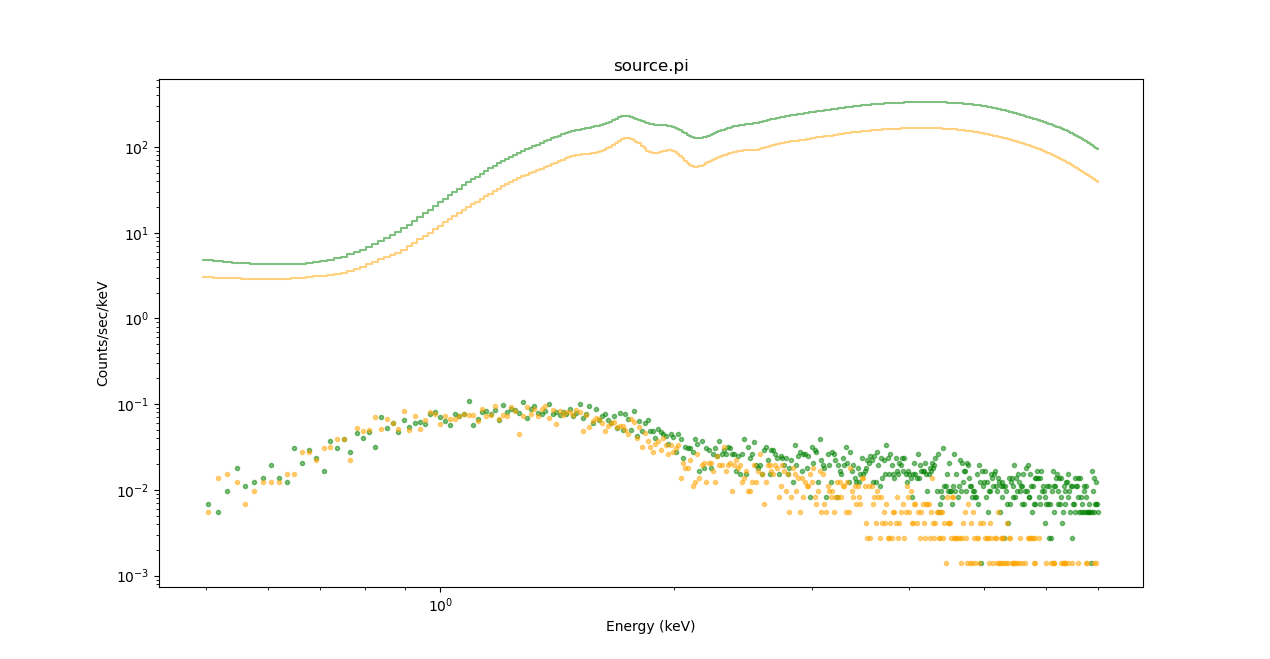### Figure 9: Plot of the ungrouped source and background data with default fits

The source region (and fit) is shown in green, the background is in orange.

The opacity of plot elements may be set with the alpha plot option.

Clearly, the default values are not a very good fit to the data. We may choose to begin the fit now, using the fit() command, or we can refine the values of the fit-by-eye, prior to fitting. By varying parameter values, while plotting and replotting the data, we can help the fitting algorithm find the best minimum. By setting the parameters to values near what we expect, we also help avoid local minima in the parameter space. (Fitting spectra is something of an art; one generally gets better fits when they have a priori knowledge of the source.) We set the values of the parameters as follows:

sherpa> a1.nH = 0.05
sherpa> b1.kT = 20
sherpa> b1.norm = 1e-02
sherpa> b2.kT = 0.5
sherpa> b2.norm.min = 1e-6
sherpa> b2.norm = 1e-05


Note that the normalization of b2, b2.norm, appears to have a better fit at values less than the default minimum. We set the b2.norm.min to a new, smaller value to give us the optimal fit. Now we have a reasonable start to the fit:

sherpa> plot("fit", "bkgfit", xlog=True, ylog=True)


The plot is visible Figure 10.

### Figure 10: Plot of the ungrouped source data with custom fits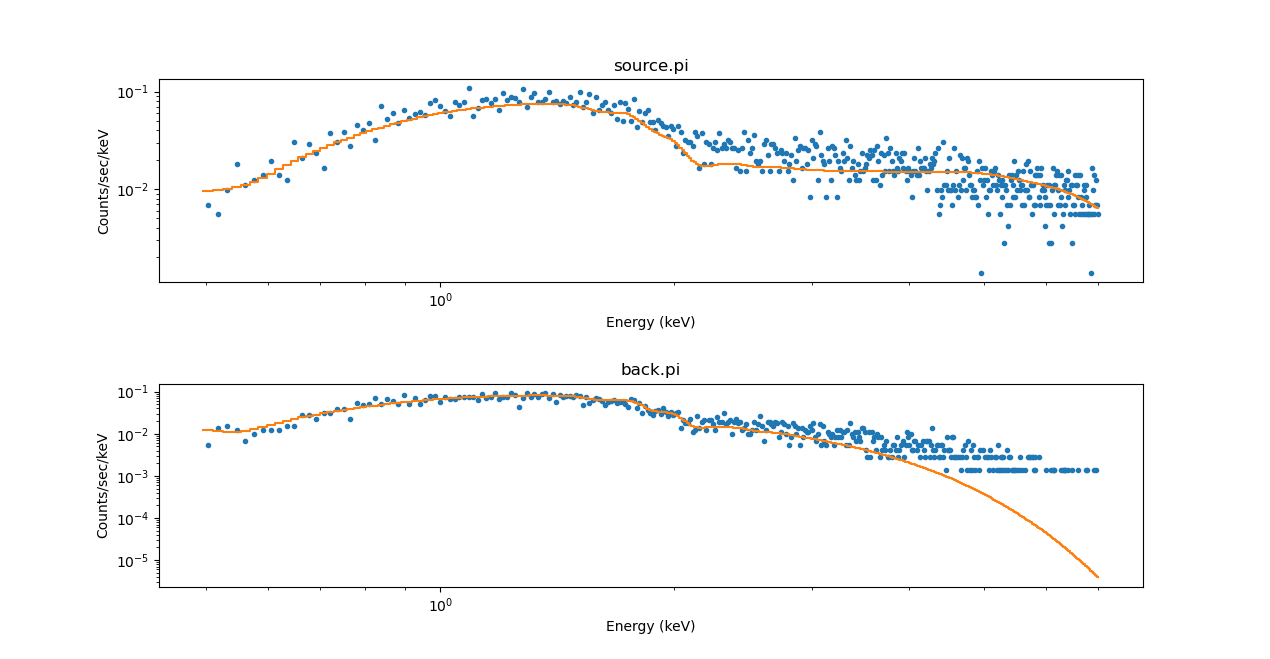### Figure 10: Plot of the ungrouped source data with custom fits

The spacing between the plots has been increased with the plt.subplots_adjust function to separate the x-axis label from the top plot and the title from the bottom plot.

In general, we may also want to change our fit statistic or optimization method with set_stat and set_method (which we have already done):

sherpa> fit()
Dataset               = 1
Statistic             = cash
Initial fit statistic = -61384.7
Final fit statistic   = -62114.5 at function evaluation 1848
Data points           = 892
Degrees of freedom    = 887
Change in statistic   = 729.847
a1.nH          0.00964099
b1.kT          5.29341
b1.norm        0.000256371
b2.kT          0.612521
b2.norm        1.27349e-05


Once the fit is complete, Sherpa will display the fit values to the screen. You may also display the overall status of the Sherpa session with the show_all and show_bkg command:

sherpa> show_all()
Data Set: 1
Filter: 0.4964-7.0080 Energy (keV)
Bkg Scale: 0.694444
Noticed Channels: 35-480
name           = source.pi
channel        = Float64
counts         = Float64
staterror      = None
syserror       = None
bin_lo         = None
bin_hi         = None
grouping       = None
quality        = None
exposure       = 49429.233467924
backscal       = 1.872535141462e-05
areascal       = 1.0
grouped        = False
subtracted     = False
units          = energy
rate           = True
plot_fac       = 0
response_ids   = 
background_ids = 

RMF Data Set: 1:1
name     = source.rmf
detchans = 1024
energ_lo = Float64
energ_hi = Float64
n_grp    = UInt64
f_chan   = UInt32
n_chan   = UInt32
matrix   = Float64
offset   = 1
e_min    = Float64
e_max    = Float64
ethresh  = 1e-10

ARF Data Set: 1:1
name     = source.arf
energ_lo = Float64
energ_hi = Float64
specresp = Float64
bin_lo   = None
bin_hi   = None
exposure = 49470.94775939
ethresh  = 1e-10

Background Data Set: 1:1
Filter: 0.4964-7.0080 Energy (keV)
Noticed Channels: 35-480
name           = back.pi
channel        = Float64
counts         = Float64
staterror      = None
syserror       = None
bin_lo         = None
bin_hi         = None
grouping       = None
quality        = None
exposure       = 49429.233467924
backscal       = 2.6964506037052e-05
areascal       = 1.0
grouped        = False
subtracted     = False
units          = energy
rate           = True
plot_fac       = 0

Background RMF Data Set: 1:1
name     = back.rmf
detchans = 1024
energ_lo = Float64
energ_hi = Float64
n_grp    = UInt64
f_chan   = UInt32
n_chan   = UInt32
matrix   = Float64
offset   = 1
e_min    = Float64
e_max    = Float64
ethresh  = 1e-10

Background ARF Data Set: 1:1
name     = back.arf
energ_lo = Float64
energ_hi = Float64
specresp = Float64
bin_lo   = None
bin_hi   = None
exposure = 49470.94775939
ethresh  = 1e-10

Model: 1
apply_rmf(apply_arf((49429.233467924 * ((xsphabs.a1 * xsbbody.b1) + (0.694444444444465 * (xsphabs.a1 * xsbbody.b2))))))
Param        Type          Value          Min          Max      Units
-----        ----          -----          ---          ---      -----
a1.nH        thawed   0.00964099            0        1e+06 10^22 atoms / cm^2
b1.kT        thawed      5.29341       0.0001          200        keV
b1.norm      thawed  0.000256371            0        1e+24
b2.kT        thawed     0.612521       0.0001          200        keV
b2.norm      thawed  1.27349e-05        1e-06        1e+24

name         = simplex
ftol         = 1.1920928955078125e-07
maxfev       = None
initsimplex  = 0
finalsimplex = 9
step         = None
verbose      = 0
reflect      = True

Statistic: Cash
Poisson Log-likelihood function.

Counts are sampled from the Poisson distribution, and so the best
way to assess the quality of model fits is to use the product of
individual Poisson probabilities computed in each bin i, or the
likelihood L:

L = (product)_i [ M(i)^D(i)/D(i)! ] * exp[-M(i)]

where M(i) = S(i) + B(i) is the sum of source and background model
amplitudes, and D(i) is the number of observed counts, in bin i.

The Cash statistic _ is derived by (1) taking the logarithm of
the likelihood function, (2) changing its sign, (3) dropping the
factorial term (which remains constant during fits to the same
dataset), and (4) multiplying by two:

C = 2 * (sum)_i [ M(i) - D(i) log M(i) ]

The factor of two exists so that the change in cash statistic from
one model fit to the next, (Delta)C, is distributed approximately
as (Delta)chi-square when the number of counts in each bin is
high.  One can then in principle use (Delta)C instead of
(Delta)chi-square in certain model comparison tests. However,
unlike chi-square, the cash statistic may be used regardless of
the number of counts in each bin.

The magnitude of the Cash statistic depends upon the number of
bins included in the fit and the values of the data
themselves.  Hence one cannot analytically assign a
goodness-of-fit measure to a given value of the Cash statistic.
Such a measure can, in principle, be computed by performing
Monte Carlo simulations. One would repeatedly sample new
datasets from the best-fit model, fit them, and note where the
observed Cash statistic lies within the derived distribution
of Cash statistics. Alternatively, the cstat statistic can
be used.

Notes
-----
The background should not be subtracted from the data when this
statistic is used.  It should be modeled simultaneously with the
source.

The Cash statistic function evaluates the logarithm of each data
point. If the number of counts is zero or negative, it's not
possible to take the log of that number. The behavior in this case
is controlled by the truncate and trunc_value settings in the
.sherpa.rc file:

- if truncate is True (the default value), then
log(trunc_value) is used whenever the data value is <= 0.  The
default is trunc_value=1.0e-25.

- when truncate is False an error is raised.

References
----------

..  "Parameter estimation in astronomy through application of
the likelihood ratio", Cash, W. 1979, ApJ 228, 939

Fit:Dataset               = 1
Statistic             = cash
Initial fit statistic = -61384.7
Final fit statistic   = -62114.5 at function evaluation 1848
Data points           = 892
Degrees of freedom    = 887
Change in statistic   = 729.847
a1.nH          0.00964099
b1.kT          5.29341
b1.norm        0.000256371
b2.kT          0.612521
b2.norm        1.27349e-05


One may visually examine the fit with the Sherpa plot functions:

sherpa> set_xlog()
sherpa> set_ylog()
sherpa> plot_fit_resid(yerrorbars=False)
WARNING: The displayed errorbars have been supplied with the data or calculated using chi2xspecvar; the errors are not used in fits with cash
WARNING: The displayed errorbars have been supplied with the data or calculated using chi2xspecvar; the errors are not used in fits with cash


### Figure 11: Simultaneous fit of the ungrouped source data (different responses)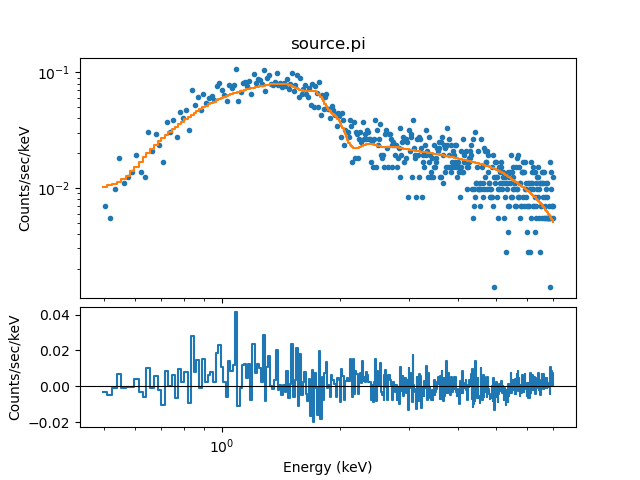### Figure 11: Simultaneous fit of the ungrouped source data (different responses)

sherpa> plot_bkg_fit_resid(yerrorbars=False)
WARNING: The displayed errorbars have been supplied with the data or calculated using chi2xspecvar; the errors are not used in fits with cash
WARNING: The displayed errorbars have been supplied with the data or calculated using chi2xspecvar; the errors are not used in fits with cash


### Figure 12: Simultaneous fit of the ungrouped background data (different responses)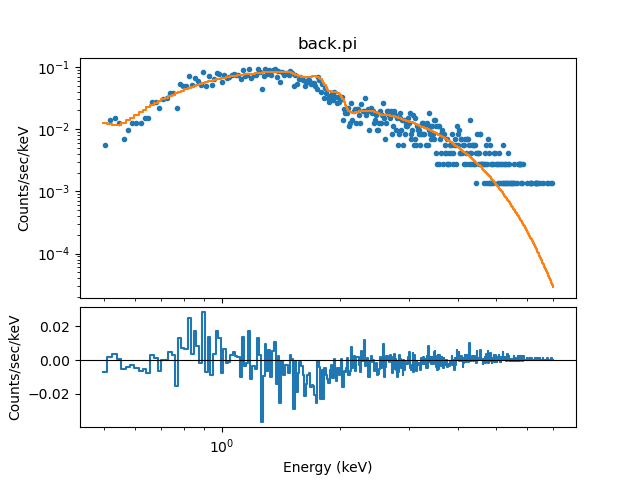### Figure 12: Simultaneous fit of the ungrouped background data (different responses)

We may also calculate the confidence 1σ error estimates on the individual parameters of the fit:

sherpa> conf()
a1.nH lower bound:      -----
a1.nH upper bound:      0.00116746
b2.norm lower bound:    -7.08686e-07
b1.norm lower bound:    -0.000162804
b2.norm +: WARNING: The confidence level lies within (1.317125e-05, 1.338944e-05)
b2.norm upper bound:    5.45475e-07
b1.kT lower bound:      -1.86173
b1.norm upper bound:    0.00117057
b2.kT lower bound:      -0.0128835
b1.kT upper bound:      4.924
b2.kT upper bound:      0.0207927
Dataset               = 1
Confidence Method     = confidence
Iterative Fit Method  = None
Statistic             = cash
confidence 1-sigma (68.2689%) bounds:
Param            Best-Fit  Lower Bound  Upper Bound
-----            --------  -----------  -----------
a1.nH          0.00964099        -----   0.00116746
b1.kT             5.29341     -1.86173        4.924
b1.norm       0.000256371 -0.000162804   0.00117057
b2.kT            0.612521   -0.0128835    0.0207927
b2.norm       1.27349e-05 -7.08686e-07  5.45475e-07


### Manually defining source and background models

We may choose to explicitly set the complete convolved model expression to be used for fitting a source data set and its associated background, using the new functions set_full_model and set_bkg_full_model, together with get_response or get_arf/get_rmf. These functions offer an alternative to using the functions set_source and set_bkg_source, which automatically convolve the appropriate instrument response with a defined source and background model expression.

The following set of commands represents the manual definition of the chosen source and background models, which were automatically (and equivalently) defined above with set_source and set_bkg_model.

sherpa> rsp = get_response()
sherpa> bkg_rsp = get_response(bkg_id=1)
sherpa> bkg_scale = get_bkg_scale(units='rate')
sherpa> src_model = rsp(xswabs.a1 * xsbbody.b1)
sherpa> bkg_model = bkg_rsp(a1 * xsbbody.b2)
sherpa> set_full_model(src_model + bkg_scale * bkg_model)
sherpa> set_bkg_full_model(bkg_model)Warning

The units argument of get_bkg_scale is used to calculate the scale factor either for background subtraction (units='counts', the default) or for background modelling (units='rate'). In this case, as we are modelling the background, we use the rate setting: for this option the ratio of the exposure times is not included in the scale factor, since the models are calculating rates and not counts.

You could also use get_arf and get_rmf in place of get_response, as follows:

sherpa> arf = get_arf()
sherpa> rmf = get_rmf()
sherpa> bgarf = get_arf(bkg_id=1)
sherpa> bgrmf = get_rmf(bkg_id=1)

sherpa> bkg_scale = get_bkg_scale(units='rate')
sherpa> src_model = rmf(arf(xswabs.a1 * xsbbody.b1))
sherpa> bkg_model = bgrmf(bgarf(a1 * xsbbody.b2))
sherpa> set_full_model(src_model + bkg_scale * bkg_model)
sherpa> set_bkg_full_model(bkg_model)


Note that the Sherpa functions which are related to a source or background model defined with set_source or set_bkg_source, such as plot_source/plot_bkg_source or calc_energy_flux, are not compatible with the complete model expression defined by the set_full_model or set_bkg_full_model functions. In order to use these Sherpa functions, users should define source and background models in the usual way with the automatic functions set_source and set_bkg_source.

## Scripting It

The file fit.py is a Python script which performs the primary commands used above; it can be executed by typing %run -i fit.py on the Sherpa command line.

The Sherpa script command may be used to save everything typed on the command line in a Sherpa session:

sherpa> script(filename="sherpa.log", clobber=False)


(Note that restoring a Sherpa session from such a file could be problematic since it may include syntax errors, unwanted fitting trials, et cetera.)

## Summary

This thread is complete, so we can exit the Sherpa session:

sherpa> quit()


## History

 14 Jan 2005 updated for CIAO 3.2: minor change in fit results 21 Dec 2005 reviewed for CIAO 3.3: no changes 01 Dec 2006 reviewed for CIAO 3.4: no changes 08 Dec 2008 updated for Sherpa 4.1 29 Apr 2009 new script command is available with CIAO 4.1.2 17 Dec 2009 updated for CIAO 4.2: the fit_bkg function is available 14 Jun 2010 updated to include confidence 1-sigma error estimates on individual parameters of fits 14 Jun 2010 updated with an example using the CIAO 4.2 Sherpa v2 functions set_full_model, set_bkg_full_model, get_response, and a new usage of the functions get_arf and get_rmf. S-Lang version of thread removed. 15 Dec 2010 updated for CIAO 4.3: new functions get_bkg_scale, calc_stat_info, and set_xlog/set_ylog are available 22 Jun 2011 renamed thread from "Independent Background Responses" to "Simultaneously Fitting Source and Background Spectra" 15 Dec 2011 reviewed for CIAO 4.4: added a warning about filtering/grouping source and background 13 Dec 2012 reviewed for CIAO 4.5: removed an outdated warning about filtering/grouping both source and background data, as the associated bug was fixed 04 Dec 2013 reviewed for CIAO 4.6: removed references to deprecated tools, updated fit results 03 Dec 2015 updated for CIAO 4.8: no content change 09 Nov 2016 reviewed for CIAO 4.9: updated fits, no content change. 25 May 2018 reviewed for CIAO 4.10: no content change; typeset equations with LaTeX. 10 Dec 2018 reviewed for CIAO 4.11, screen output revised 11 Dec 2019 Updated to use Matplotlib in CIAO 4.12 and to take advantage of changes to the plot support (e.g. plot_fit(ylog=True)); the text and commands have been revised slightly. 17 Dec 2020 Updated for CIAO 4.13: the plots now use the new style for PHA datasets; a section on grouping background and source datasets has been added; the Simultaneously Fit Source and Background with Independent Responses section now uses a Poisson-based likelihood when fitting the ungrouped data; and added discussions about changes to get_bkg_scale in CIAO 4.13. 24 Mar 2022 revised for CIAO 4.14, no new content change.